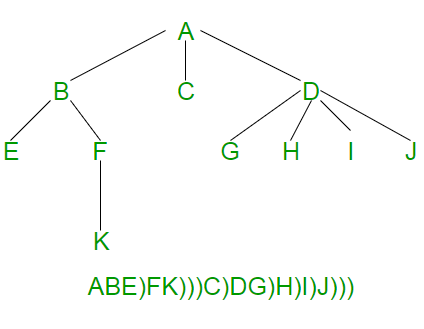# Serialize and Deserialize an N-ary Tree

Given an N-ary tree where every node has at-most N children. How to serialize and deserialze it? Serialization is to store tree in a file so that it can be later restored. The structure of tree must be maintained. Deserialization is reading tree back from file.

This post is mainly an extension of below post.
Serialize and Deserialize a Binary Tree

In an N-ary tree, there are no designated left and right children. An N-ary tree is represented by storing an array or list of child pointers with every node.
The idea is to store an ‘end of children’ marker with every node. The following diagram shows serialization where ‘)’ is used as end of children marker.Following is C++ implementation of above idea.

 `// A C++ Program serialize and deserialize an N-ary tree ` `#include ` `#define MARKER ')' ` `#define N 5 ` `using` `namespace` `std; ` ` `  `// A node of N-ary tree ` `struct` `Node { ` `   ``char` `key; ` `   ``Node *child[N];  ``// An array of pointers for N children ` `}; ` ` `  `// A utility function to create a new N-ary tree node ` `Node *newNode(``char` `key) ` `{ ` `    ``Node *temp = ``new` `Node; ` `    ``temp->key = key; ` `    ``for` `(``int` `i = 0; i < N; i++) ` `        ``temp->child[i] = NULL; ` `    ``return` `temp; ` `} ` ` `  `// This function stores the given N-ary tree in a file pointed by fp ` `void` `serialize(Node *root, ``FILE` `*fp) ` `{ ` `    ``// Base case ` `    ``if` `(root == NULL) ``return``; ` ` `  `    ``// Else, store current node and recur for its children ` `    ``fprintf``(fp, ``"%c "``, root->key); ` `    ``for` `(``int` `i = 0; i < N && root->child[i]; i++) ` `         ``serialize(root->child[i],  fp); ` ` `  `    ``// Store marker at the end of children ` `    ``fprintf``(fp, ``"%c "``, MARKER); ` `} ` ` `  `// This function constructs N-ary tree from a file pointed by 'fp'. ` `// This functionr returns 0 to indicate that the next item is a valid ` `// tree key. Else returns 0 ` `int` `deSerialize(Node *&root, ``FILE` `*fp) ` `{ ` `    ``// Read next item from file. If theere are no more items or next ` `    ``// item is marker, then return 1 to indicate same ` `    ``char` `val; ` `    ``if` `( !``fscanf``(fp, ``"%c "``, &val) || val == MARKER ) ` `       ``return` `1; ` ` `  `    ``// Else create node with this item and recur for children ` `    ``root = newNode(val); ` `    ``for` `(``int` `i = 0; i < N; i++) ` `      ``if` `(deSerialize(root->child[i], fp)) ` `         ``break``; ` ` `  `    ``// Finally return 0 for successful finish ` `    ``return` `0; ` `} ` ` `  `// A utility function to create a dummy tree shown in above diagram ` `Node *createDummyTree() ` `{ ` `    ``Node *root = newNode(``'A'``); ` `    ``root->child = newNode(``'B'``); ` `    ``root->child = newNode(``'C'``); ` `    ``root->child = newNode(``'D'``); ` `    ``root->child->child = newNode(``'E'``); ` `    ``root->child->child = newNode(``'F'``); ` `    ``root->child->child = newNode(``'G'``); ` `    ``root->child->child = newNode(``'H'``); ` `    ``root->child->child = newNode(``'I'``); ` `    ``root->child->child = newNode(``'J'``); ` `    ``root->child->child->child = newNode(``'K'``); ` `    ``return` `root; ` `} ` ` `  `// A utlity function to traverse the constructed N-ary tree ` `void` `traverse(Node *root) ` `{ ` `    ``if` `(root) ` `    ``{ ` `        ``printf``(``"%c "``, root->key); ` `        ``for` `(``int` `i = 0; i < N; i++) ` `            ``traverse(root->child[i]); ` `    ``} ` `} ` ` `  `// Driver program to test above functions ` `int` `main() ` `{ ` `    ``// Let us create an N-ary tree shown in above diagram ` `    ``Node *root = createDummyTree(); ` ` `  `    ``// Let us open a file and serialize the tree into the file ` `    ``FILE` `*fp = ``fopen``(``"tree.txt"``, ``"w"``); ` `    ``if` `(fp == NULL) ` `    ``{ ` `        ``puts``(``"Could not open file"``); ` `        ``return` `0; ` `    ``} ` `    ``serialize(root, fp); ` `    ``fclose``(fp); ` ` `  `    ``// Let us deserialize the storeed tree into root1 ` `    ``Node *root1 = NULL; ` `    ``fp = ``fopen``(``"tree.txt"``, ``"r"``); ` `    ``deSerialize(root1, fp); ` ` `  `    ``printf``(``"Constructed N-Ary Tree from file is \n"``); ` `    ``traverse(root1); ` ` `  `    ``return` `0; ` `}`

Output:

```Constructed N-Ary Tree from file is
A B E F K C D G H I J```

The above implementation can be optimized in many ways for example by using a vector in place of array of pointers. We have kept it this way to keep it simple to read and understand.

Attention reader! Don’t stop learning now. Get hold of all the important DSA concepts with the DSA Self Paced Course at a student-friendly price and become industry ready.

My Personal Notes arrow_drop_up
Article Tags :
Practice Tags :

7

Please write to us at contribute@geeksforgeeks.org to report any issue with the above content.